# Calculating Velocity Problem 7 59 Riding a bicycle

• Slides: 10
Download presentationCalculating Velocity Problem 7. 59* Riding a bicycle into headwind By: Andrew Quinn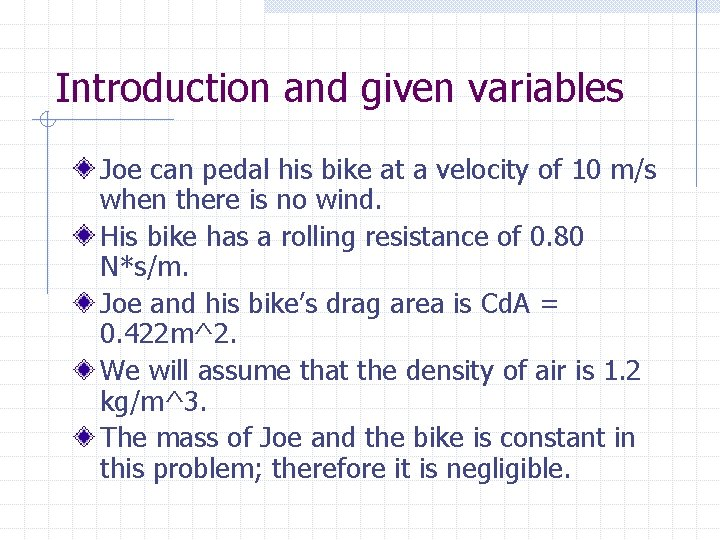Introduction and given variables Joe can pedal his bike at a velocity of 10 m/s when there is no wind. His bike has a rolling resistance of 0. 80 N*s/m. Joe and his bike’s drag area is Cd. A = 0. 422 m^2. We will assume that the density of air is 1. 2 kg/m^3. The mass of Joe and the bike is constant in this problem; therefore it is negligible.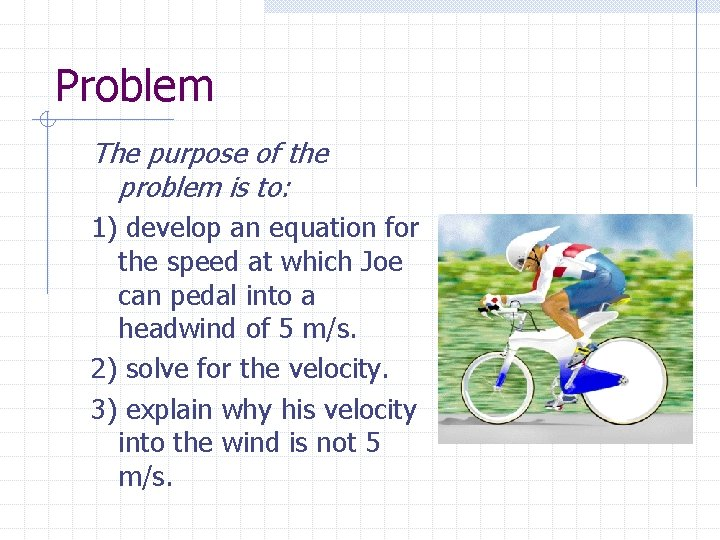Problem The purpose of the problem is to: 1) develop an equation for the speed at which Joe can pedal into a headwind of 5 m/s. 2) solve for the velocity. 3) explain why his velocity into the wind is not 5 m/s.Step 1: Calculate his force. First of all, we must calculate the amount of force that Joe is exerting when there is no wind That force is equal to the frictional drag of him and his bike while riding in addition to the friction due to the rolling resistance of his bike.Calculations We can calculate the drag of Joe and his bike by using the following equation Eq. 7. 62 CD = drag/ (1/2*r*V^2*A) Rearranging this equation, we solve for the drag. Drag = CDA*1/2*r*V^2 Substituting the given values, we find that the drag = 25. 32 NewtonsCalculations (continued) Next, we calculate the friction due to the rolling resistance of the bike. This is done by multiplying the rolling resistance by the velocity. Friction due to rolling resistance = 0. 8 N*s/m * 10 m/s = 8 Newtons This value is added to the drag calculated earlier (25. 32 Newtons) to determine the total force he is overcoming which equals 33. 32 Newtons.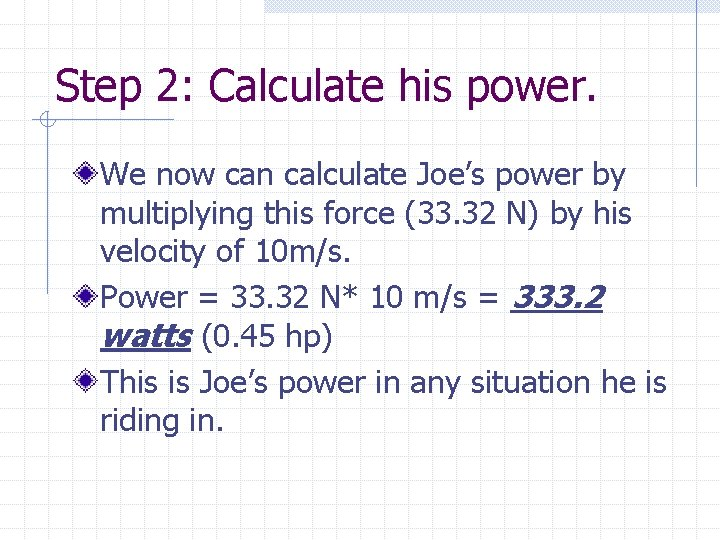Step 2: Calculate his power. We now can calculate Joe’s power by multiplying this force (33. 32 N) by his velocity of 10 m/s. Power = 33. 32 N* 10 m/s = 333. 2 watts (0. 45 hp) This is Joe’s power in any situation he is riding in.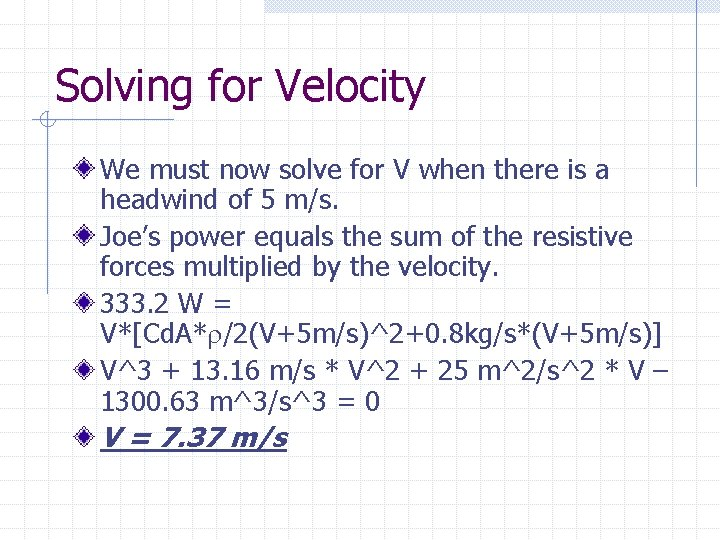Solving for Velocity We must now solve for V when there is a headwind of 5 m/s. Joe’s power equals the sum of the resistive forces multiplied by the velocity. 333. 2 W = V*[Cd. A*r/2(V+5 m/s)^2+0. 8 kg/s*(V+5 m/s)] V^3 + 13. 16 m/s * V^2 + 25 m^2/s^2 * V – 1300. 63 m^3/s^3 = 0 V = 7. 37 m/s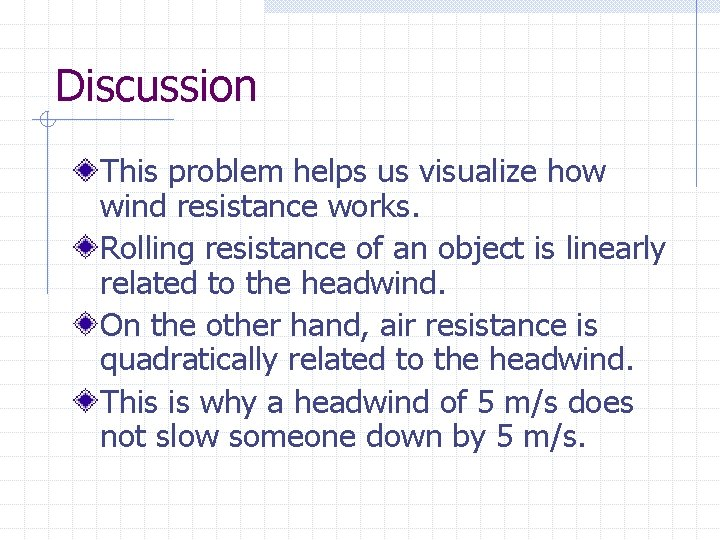Discussion This problem helps us visualize how wind resistance works. Rolling resistance of an object is linearly related to the headwind. On the other hand, air resistance is quadratically related to the headwind. This is why a headwind of 5 m/s does not slow someone down by 5 m/s.Biomedical Application It is hard to relate this problem to a biomedical application inside the body. However with this information, we can see how one can calculate the endurance of a biker by knowing the current winds that he is riding in. A biker’s energy level is an important factor to consider when monitoring his health.